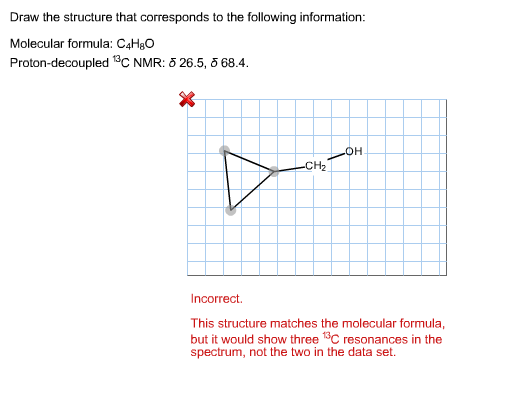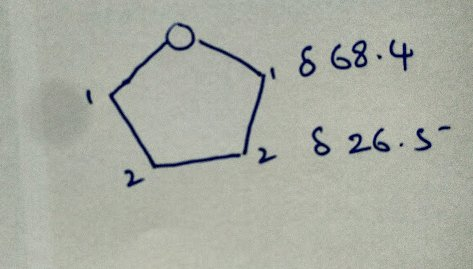# Question & Answer: Draw the structure that corresponds to the following information: Molecular formula…..Draw the structure that corresponds to the following information: Molecular formula: C_4H_8O Proton-decoupled ^13 C NMR: delta 26.5, delta 68.4. Incorrect. This structure matches the molecular formula, but it would show three ^13 C resonances in the spectrum, not the two in the data set.

Molecular formula = C4H8O
Since the formula is CnH2nO, the molecule should have one double bond or it should be a cyclic compound.

Don't use plagiarized sources. Get Your Custom Essay on
Question & Answer: Draw the structure that corresponds to the following information: Molecular formula…..
GET AN ESSAY WRITTEN FOR YOU FROM AS LOW AS \$13/PAGE

13CNMR = 26.5, 68.4

Since we have only two peaks in the 13C NMR, the molecule contains chemically two different carbon atoms.
Ether carbon, show a chemical shift at approx. 68, the molecule could be ether molecule.

Therefore, the only option will be the molecule should be a cyclic ether.
Therefore, the molecule is Tetrahydrofuran